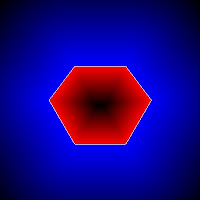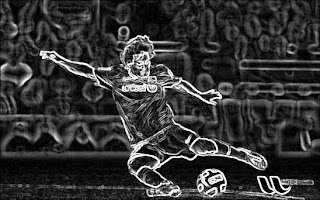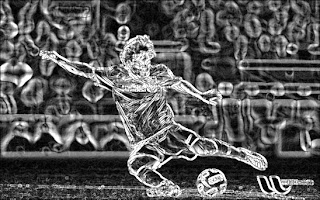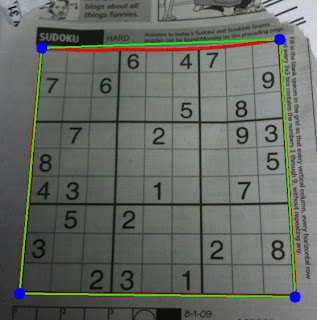# OpenCV-Python

### OpenCV Python Tutorials

opencvpython.blogspot.com

## Fast Array Manipulation in Numpy

Hi,

This post is to explain how fast array manipulation can be done in Numpy. Since we are dealing with images in OpenCV, which are loaded as Numpy arrays, we are dealing with a little big arrays. So we need highly efficient method for fast iteration across this array.

For example, consider an image of size 500x500. If we want to access all the pixels, this itself becomes 250000 calculations. To deal with this, Numpy has got some pretty cool methods. I will explain two of them here, which I know.

For this, I take an example case: You have a 500x500 numpy array of random integers between 0 and 5, ie only 0,1,2,3,4 (just consider you got it as a result of some calculations). These integers actually correspond to different colors like below:

0 ---> Green, [0,255,0]
1 ---> Blue, [255,0,0] // Note that according to OpenCV standards, it is BGR, not RGB
2 ---> Red , [0,0,255]
3 ---> White, [255,255,255]
4 ---> Black, [0,0,0]

So you want to create another 500x500x3 array (or a color image) where integers in x is replaced by corresponding color value.

First of all we deal with our normal method, which is direct indexing method.

What we normally do? Yes, a double loop.

Method 1 : Direct element access
`for i in x.rows:    for j in x.cols:        check what value at x[i,j]        put corresponding color in y[i,j]`
So that is given below:

First create necessary data, input array 'x', output array 'y', colors etc.

`import numpy as npimport timex = np.random.randint(0,5,(500,500))green = [0,255,0]blue = [255,0,0]red = [0,0,255]white = [255,255,255]black = [0,0,0]rows,cols = x.shapey = np.zeros((rows,cols,3),np.uint8)  # for output`

Now enter the loop:

`for i in xrange(rows):    for j in xrange(cols):        k = x[i,j]        if k==0:            y[i,j] = green        elif k==1:            y[i,j] = blue        elif k==2:            y[i,j] = red        elif k==3:            y[i,j] = white        else:            y[i,j] = black`

It took about 40-50 seconds to finish the work (I am considering only the loop, and the time depends on the system configuration. So better check at the comparison of results).

Method 2 : Using item() and itemsize()

We normally use k = x[i,j] or x[i,j] = k to read or write an array element. It is very simple, good for large arrays at a single step.

But this style is not at all good for cases like above, where, out of 250000 elements, select each one and modify each one separately. For that, Numpy has got a method to use, ie x.item() to access an element and x.itemset() to write an element. They are much faster than direct accessing. So next we implement our problem using these features ( Only loop portion is given, all others are same):

`for i in xrange(rows):    for j in xrange(cols):        k = x.item(i,j)        if k==0:                        y1.itemset((i,j,0),green)            y1.itemset((i,j,1),green)            y1.itemset((i,j,2),green) elif k==1:            y1.itemset((i,j,0),blue)            y1.itemset((i,j,1),blue)            y1.itemset((i,j,2),blue)        elif k==2:            y1.itemset((i,j,0),red)            y1.itemset((i,j,1),red)            y1.itemset((i,j,2),red)        elif k==3:            y1.itemset((i,j,0),white)            y1.itemset((i,j,1),white)            y1.itemset((i,j,2),white)                    else:            y1.itemset((i,j,0),black)            y1.itemset((i,j,1),black)            y1.itemset((i,j,2),black) `

(Don't be disappointed at the length of code, you will be happy when you see the performance.)

This method took nearly 5 seconds to complete the task. On my calculations, it is around 9-10x faster than the previous method. And that is good result, although length of code is a little problem.

But wait, there is a third method, called palette method.

Method 3 : Palette method

Here, there is no loop. Just three lines of code:

`color = [green,blue,red,white,black]color = np.array(color,np.uint8)y = color[x]`

Finished. See, you can considerably reduce the size of code a lot. And what about performance ? It took less than 0.2 seconds. Just compare the results:

Compared to first method, it is around 350x faster.
Compared to second method, it is around 30-40x faster.

Isn't it good, Reducing the code size to 3 lines, while speeding up the method by more than 300 times? (Truly saying, even I was shocked seeing the performance. I knew it would increase the speed, but never thought this much).

So, to understand what palette methods does and how to utilize it in image processing, we take another experiment with small sample of size 3x3.

Fist take an array of size 3x3 and elements includes only digits (0-9):

`>>> a = np.random.randint(0,10,(3,3))>>> aarray([[9, 8, 4],       [9, 0, 8],       [6, 6, 3]])`

Next we make another array 'b'. ( You can consider it as the color array).

What should be its rows? It depends on how many color you need. In this example, 'a' has only 9 type of elements (ie digits from 0 to 9) and each corresponds to a color. So we need 9 rows here.

And how many columns ? Are you going for RGB color? Then let there be 3 columns. Or grayscale intensity? Then only one column is sufficient. Here, I take grayscale, so single column,or just an 1-dimensional array.

`>>> b = np.random.randint(0,255,10)>>> barray([ 97, 177, 237,  29,  51, 230,  92, 198,   6,   7])`

See, b = 7. That exactly is happening in palette method. When you type b[a], it actually implies b[i for i in a], ie it takes each element of 'a' and subtitute for 'a' in b[a].

So what ? In our case, when we give c = b[a], it means, c[0,0] = b[ a[0,0] ], ie c[0,0] = b = 7, since a[0,0]=9.
Similarly c[0,1] = b[ a[0,1] ]  ==> c[0,1] = b = 6, and so on. So final result is as follows:

`>>> c = b[a]>>> carray([[ 7,  6, 51],       [ 7, 97,  6],       [92, 92, 29]])`

ie, replace every element in 'a' with element in 'b', of which index is decided by the value in 'a'.

Now we need a practical example from image processing. Best example is the PointPolygonTest in OpenCV. First, learn and understand the PointPolygonTest code.

That code, on running, took a minimum of 4.084 seconds (out of 10 runs). Now I removed the part under line 39 in that code and added code as follows, which is a implementation of palette method:

First rounded the values of 'res' to nearest integer.
`res = np.int0(np.around(res))`
Later, found minimum value in it and multiplied it with 255. Same with maximum also. They are to be used in calculation of color.

`mini = res.min()minie = 255.0/minimaxi = res.max()maxie = 255.0/maxi`

Now create the image to draw the output. Remember, rows = maximum distance - minimum distance + 1 & columns = 3, for RGB values.
`drawing = np.zeros((maxi-mini+1,3),np.uint8)`

Now we add minimum distance to the 'res'. It is because, some values in 'res' are negative (distance to point outside contour). So when we apply palette method, negative values will be taken as indices which are not allowed. For that, we add minimum value to all elements in 'res', so that, in new 'res', minimum value is 0.

`res = res+abs(mini)`

Next part we define the color. For that, we need a single loop, which iterates all the values between res.minimum(mini) and res.maximum(maxi). So, instead of iterating over 160000 values in original method, we just iterate over only less than 300 values (in this case, maxi-mini ≈ ≈ 300). Then coloring scheme is same as in previous method.

`for h,i in enumerate(xrange(mini,maxi+1)):    if i<0:        drawing.itemset((h,0),255-int(minie*i))    elif i>0:        drawing.itemset((h,2),255-int(maxie*i))    else:        drawing[h]=[255,255,255]`

Now finally apply the palette.

`d = drawing[res]`

This method took a maximum time of 0.08 seconds (out of 10 runs). That means, it increases the speed by more than 50X. That is a good improvement.

Finally, in this case, although both output look similar, they are not identical. There may be small variations due to rounding off. But it is just a shift of only one pixel and won't be a problem. Look at the results below:Results in palette method.Result in normal method

See any difference between both results ? ( If any, it will be negligible compared to performance)

Hope, you enjoyed it. Let me have your feedback.

Regards,
ARK

## Image Derivatives and its Applications

Hi,

You can find image derivatives using cv2.Sobel() and cv2.Scharr() functions in OpenCV. There is a nice tutorial and explanation about this in OpenCV site, "Sobel Derivatives". You can find a Python adaptation here:  sobel.py

This post is written to show you some of those functions.This is the original image →First I applied Sobel derivatives in vertical and horizontal directions and blended them with equal weights, 0.5. Here is the result →Next, instead of blending, I directly added them. It gives you a much more bright result, just a fancy development, nothing special →Next, I applied Scharr instead of Sobel, and again blended them. Here is the result →

Scharr output is considered to be much more accurate.Next I applied Laplacian operator to the same image. It is sum of second derivatives in both the directions. If you use Sobel to find second derivative and take their sum, you get almost same result.

You can find tutorial about laplacian operator here: Laplace Operator. You can find corresponding Python implementation here : Python CodeFinally, there is Canny edge detector. Here is the result for canny edge detector for a low threshold value of 74. Original image and edge image is bitwise_and operated to make image a little colorful.

You can find tutorial about canny edge detector here : Canny Edge Detector. Its corresponding Python code is here : Python code

With Regards,
ARK

## Sudoku Solver - Part 2

Hi,

This is the continuation of the article : Sudoku Solver - Part 1

So we start implementing here.

Below is the image I used to work with.Original  Image
So, first we import necessary libraries.

`import cv2import numpy as np`

Then we load the image, and convert to grayscale.

`img =  cv2.imread('sudoku.jpg')gray = cv2.cvtColor(img,cv2.COLOR_BGR2GRAY)`

Image Pre-processing :

I have done just noise removal and thresholding. And it is working. So I haven't done anything extra.

`gray = cv2.GaussianBlur(gray,(5,5),0)thresh = cv2.adaptiveThreshold(gray,255,1,1,11,2)`

Below is the result :Result of adaptive thresholding
Now two questions may arise :

1) What is the need of smoothing here?
2) Why Adaptive Thresholding ? Why not normal Thresholding using cv2.threshold()  ?

Find the answers here : Some Common Questions

Find Sudoku Square and Corners :

Now we find the sudoku border. For that, we are taking a practical assumption : The biggest square in the image should be Sudoku Square. In short, image should be taken close to Sudoku, as you can see in the input image of demo.

So a lot of things are clear from this : Image should have only one square, Sudoku Square, or not, Sudoku Square must be the biggest. If this condition is not true, method fails.

It is because, we find the sudoku square by finding the biggest blob ( an independant particle) in the image. So if biggest blob is something other than Sudoku, that blob is processed. So, I think you will keep an eye on it.

We start by finding contours in the thresholded image:

`contours, hierarchy = cv2.findContours(thresh, cv2.RETR_TREE, cv2.CHAIN_APPROX_SIMPLE)`

Now we find the biggest blob, ie blob with max. area.

For this, first we find area of each blob. Then we filter them by area. We consider a blob for next processing only if its area is greater than a particular value (here, it is 100). If so, we approximate the contours. It removes unwanted coordinate values in the contour and keep only the corners. So if number of corners equal to four, that is a square (actually, a rectangle). If it has the maximum area among all detected squares, it is out Sudoku square.

`biggest = Nonemax_area = 0for i in contours:        area = cv2.contourArea(i)        if area > 100:                peri = cv2.arcLength(i,True)                approx = cv2.approxPolyDP(i,0.02*peri,True)                if area > max_area and len(approx)==4:                        biggest = approx                        max_area = area`

For you to understand between original contour and approximated contour, I have drawn it on the image (using cv2.drawContours() function). Red line is the original contour, Green line is the approximated contour and corners marked in blue color circles.Border and corners detected
Look at the top edge of sudoku. Original contour ( Red line) grazes on the edge of square and it is curved. Approximated contour ( Green line) just made it into a straight line.

Now, a simple question may arise. What is the benefit of filtering contours with respect to area? What is the need of removing them ? In simple words, it is done for speed up of the program. Although it may give you a little performance ( in the range of few milliseconds), even that will be good for those who want to implement it in real time. For more explanation, visit : Some Common Questions

Summary :

So, in this section, we have found the boundary of sudoku. Next part is the image transformation. I will explain it in next post.

Until then, I would like to know your feedback, doubts etc.

With Regards
ARK

## Sudoku Solver - Some Common Questions

Hi,

This is a post to answer some common questions that can arise while dealing with the Sudoku Solver.

Question 1 : What is the need of Smoothing?

Answer : You will understand its need if you see the result without applying Smoothing. Below is the result of Adaptive Threshold without Smoothing.Result of adaptive noise without smoothing
You can see the same result after applying a smoothing:After smoothing
Compare the results. There are lot of noises in the first case. So we have to remove them in the next step which is an extra task.

I just compared number of independent objects found (ie contours ) in both the cases. Below is the result:

First without smoothing:
`>>> len(contours)3109`

Next after smoothing:
`>>> len(contours)450`

See the difference. Without smoothing, we are dealing with 7 times the number of objects than those found after smoothing. So which one is good?

To know different Smoothing Techniques : Smoothing Techniques in OpenCV

Question 2 : Why adaptive thresholding ? Why not normal thresholding ?

AnswerReason, You will understand when we compare the results of them.

Below is the result, I got using Adaptive Threshold :Result of Adaptive Threshold
Now we apply normal thresholding for a value of 96 ( 96 is the auto threshold value generated by GIMP):Normal thresholding for value = 96
Now see the difference. It is because normal thresholding thresholds the image taken as a whole, while adaptive threshold thresholds the image taking an optimum value for a local neighbourhood.

To know more about thresholding techniques :

Question 3 What is the benefit of filtering contours with respect to area?

Answer : 1) To avoid small noises which has an area less than prescribed value and we are sure it can't be the square

2) It also improves the speed a little bit.

I will show you some performance comparisons below:

A)  We have already calculated number of objects (contours) found, which is 450. Without having any area filter, it process all the 450 contours. For that, you can just change the code as below:

`for i in contours:    if area > min_size:        peri = cv2.arcLength(i,True)        approx = cv2.approxPolyDP(i,0.02*peri,True)        if area > max_area and len(approx)==4:            biggest = approx            max_area = area`

It checks all the 450 contours for maximum area and it takes an average of 30 ms.

B)  Now we implement a filter for area of 100, as explained in the original code. Then it takes checks only 100 contours and takes only an average of 15 ms. So we get 2X performance.

C)  Now change the value from 100 to 1/4 of the image size. Check the code below:

`min_size = thresh.size/4for i in contours:    if area > min_size:        peri = cv2.arcLength(i,True)        approx = cv2.approxPolyDP(i,0.02*peri,True)        if area > max_area and len(approx)==4:            biggest = approx            max_area = area`

Now it checks only one contour,our square, and takes only an average of 3 ms. ie, 10X performance.

Now, although time difference is only 27 ms, it will be highly useful if we implement it in real time.

So, it all depends on how you use it.

## Smoothing Techniques in OpenCV

Hi,

This post is an additional note to official OpenCV tutorial : Smoothing Images

( Its corresponding Python code can be found here : smoothing.py )

Below I would like to show you the results I got when I applied four smoothing techniques in OpenCV, ie cv2.blur, cv2.GaussianBlur, cv2.medianBlur and cv2.bilateralFilter. Kernel size, I used in all cases were 9. See the result below :

Original Image:Original Image
After Homogeneous Blur, cv2.blur() :Result of blurring
After Gaussian Blur , cv2.GaussianBlur():Result of Gaussian Filter
It is much more clear than previous.

After median blur, cv2.medianBlur() :After median blur
It has become somewhat like a painting. See eye, it has become completely black.

Finally, after bilateral filter :

This result has high similarity with original image. It is because, it doesn't smooth the edge, instead smooth small noises leaving edges same way. So to see difference, zoom image to left face and check carefully. Then you will understand, face part will have become much more smoother, in short, much more glamorous. There is a nice explanation of bilateral filter at this link : Bilateral Filtering.

But the main problem is that, it takes more time than other filters.

Regards,
ARK

## Difference between Matrix Arithmetic in OpenCV and Numpy

Hi,

This is a small post to show you an important difference in arithmetic operations in OpenCV and Numpy.

As an example,  I take addition as operation.

As you know, images are loaded in OpenCV as "uint8" data. ie 8 bit data. So all the values in the matrix (or image) lie between 0 and 255.

So, even if you add or subtract two numbers, result lies between 0 and 255.

For eg,      255+1 ≠ 256  for 'uint8' data

So what is the answer in above case?

There lies the difference between OpenCV and Numpy. I will demonstrate it using Python terminal.

First create two datas of uint8 type, x = 255, y = 1

`>>> x = np.array(,np.uint8)>>> y = np.array(,np.uint8)`

OpenCV

`>>> cv2.add(x,y)array([], dtype=uint8)`

ie 255+1 = 255 in OpenCV. It is because arithmetic operations in OpenCV are clipped or saturated operations. ie , they clip values wrt data type. If uint8, it clips all values 0 and 255. So if you add two gray pixels, a = 127 and b = 129, you get c = 255, a white pixel, which is OK and necessary in Image Processing

Numpy

Now we add x and y in Numpy.

`>>> x+yarray(, dtype=uint8)`

ie 255+1 = 0 in Numpy. It is because Numpy performs a modulo-256 operation. So 256 % 256 = 0.

But what it implies in image processing? If you add a value of  '1' to a white pixel, you get a pure black pixel, which is completely unfavorable in image processing. If you add a = 127 and b = 128, again you get a black pixel.

So better stick to OpenCV functions for image arithmetic operations.

Regards,
ARK

## Sudoku Solver - Part 1

Hi,

Now I would like to post a series of tutorials on "Sudoku Solver" .

Actually I started this a few months ago, but got stuck at final part, more specifically, the OCR part. But after a little hacks, I could find a simple method for OCR using kNN. Hope you have read that article  !!!

In this post, I will tell you what exactly I did to develop a "Sudoku Solver".

What exactly it does?

This project on successful completion, accept an image of Sudoku as input, and returns a solved Sudoku back.

See a demonstration below:Output of sudoku solverInput Image.

How to accomplish this :

It can be done implementing the methods given in image below :

We will deal with each of one of the steps above:
1. Reading the Image : It is our normal image reading in OpenCV
2. Image Pre-processing : It includes noise removal, brightness/contrast adjustment, thresholding etc.
3. Find Sudoku Square & Corners : Here we find outer border of Sudoku square and its corners.
4. Image Transformation : Here we reshape irregular Sudoku in input image to a perfect square.
5. Recognize the digit (OCR) : Recognizes the digits in input image and place them in correct position
6. Solve the Sudoku : Here, real solving of Sudoku take place.
7. Project back the Result : We project the solved Sudoku to image as shown in demo.
In some steps, we take some practical assumptions. One, I would like to tell you now :

The biggest square in the image should be Sudoku Square. In short, image should be taken close to Sudoku, as you can see in the input image of demo. ( Reason, I will tell in upcoming posts).

That is all the theory about this. From next post onwards, we get into practicals on how to implement this.

ARK.

Inspired by
1 - Google Goggles Android Application
2 - C++ implementation of Sudoku Solver at Aishack.in
And more...

## Barcode Detection

Hi,

In this post, I would like to share a simple method on how to detect barcode in an image.

Method :

1) Convert image to grayscale, let it be 'img'

2) Now find derivative of the image in both horizontal and vertical direction, let them be 'imgx' and 'imgy' respectively.
imgx = d (img) / dx

imgy = d (img) / dy

3) Now subtract 'imgy' from 'imgx'.
res = imgx - imgy

Peculiarity of barcode is that, it has high gradient in horizontal direction, and low gradient in vertical direction. So their difference gives maximum value at barcode region

Implementation Results:result 1result 2

It is just a basic implementation. So in case of noise or other problems, additional preprocessing methods should be done.

Another problem is the rotation of the barcode. It works well only if barcode is horizontal. Otherwise, other preprocessing methods should be done to make barcode horizontal.

Code :

I have shared the code for this in an answer to a question on stackoverflow.com. Please visit the page.

With Regards
ARK.

## Skeletonization using OpenCV-Python

I see people asking an algorithm for skeletonization very frequently. At first, I had no idea about it. But today, I saw a which demonstrates simple method to do this. Code was in C++, so I would like to convert it to Python here.

What is Skeletonization?

Answer is just right in the term. Simply, it make a thick blob very thin, may be one pixel width. Visit the wikipedia page for more details : Topological Skeleton

Code :

`import cv2import numpy as npimg = cv2.imread('sofsk.png',0)size = np.size(img)skel = np.zeros(img.shape,np.uint8)ret,img = cv2.threshold(img,127,255,0)element = cv2.getStructuringElement(cv2.MORPH_CROSS,(3,3))done = Falsewhile( not done):    eroded = cv2.erode(img,element)    temp = cv2.dilate(eroded,element)    temp = cv2.subtract(img,temp)    skel = cv2.bitwise_or(skel,temp)    img = eroded.copy()    zeros = size - cv2.countNonZero(img)    if zeros==size:        done = Truecv2.imshow("skel",skel)cv2.waitKey(0)cv2.destroyAllWindows()`

Below is the result I got:References :

1) http://felix.abecassis.me/2011/09/opencv-morphological-skeleton/

## Detecting Glass in OpenCV

OpenCV developers are busy in implementing "Detection of Glass". And it seems they are almost finished with this.

It is implementation of "A Geodesic Active Contour Framework for Finding Glass" by K. McHenry and J. Ponce, CVPR 2006. (Download paper)

You can visit their meeting notes for implementation progress.

OpenCV Meeting Notes Minutes 2012-03-05

OpenCV Meeting Notes Minutes 2012-02-21

OpenCV Meeting Notes Minutes 2012-04-24

With Regards

ARK

## Report "OpenCV-Python"

Are you sure you want to report this post for ?

Cancel
×